+

# Profitability Ratios

Author: Sophia Tutorial
##### Description:

Determine a company's operating margin, profit margin, ROA, or ROE.

(more)### Developing Effective Teams

*No strings attached. This college course is 100% free and is worth 1 semester credit.

46 Sophia partners guarantee credit transfer.

299 Institutions have accepted or given pre-approval for credit transfer.

* The American Council on Education's College Credit Recommendation Service (ACE Credit®) has evaluated and recommended college credit for 33 of Sophia’s online courses. Many different colleges and universities consider ACE CREDIT recommendations in determining the applicability to their course and degree programs.

Tutorial

what's covered
In this lesson, you will learn about a company's operating margin, net and gross profit margins, ROA, and ROE. Specifically, this lesson will cover:
1. Operating Margin Ratio
2. Profit Margin Ratio
3. Return on Assets (ROA) Ratio
4. Basic Earning Power (BEP) Ratio
5. Return on Equity (ROE) Ratio

## 1. Operating Margin Ratio

The financial job of a company is to earn a profit, which is different than earning revenue. If a company doesn’t earn a profit, their revenues aren’t helping the company grow. It is not only important to see how much a company has sold, it is important to see how much a company is making.

The operating margin ratio (also called the operating profit margin or return on sales) is a ratio that shines a light on how much money a company is actually making in profit. It is found by dividing operating income by revenue, where operating income is revenue minus operating expenses.

formula

Operating Margin Ratio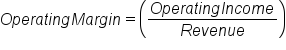The higher the ratio is, the more profitable the company is from its operations.

EXAMPLE

An operating margin of 0.5 means that for every dollar the company takes in revenue, it earns \$0.50 in profit. A company that is not making any money will have an operating margin of 0; it is selling its products or services but isn’t earning any profit from those sales.

However, the operating margin is not a perfect measurement. It does not include things like capital investment, which is necessary for the future profitability of the company. Furthermore, the operating margin is simply revenue. That means that it does not include things like interest and income tax expenses. Since non-operating incomes and expenses can significantly affect the financial well-being of a company, the operating margin is not the only measurement that investors scrutinize. The operating margin is a useful tool for determining how profitable the operations of a company are, but not necessarily how profitable the company is as a whole.

terms to know

Operating Margin Ratio
A ratio that determines how much money a company is actually making in profit.
Operating Income
Equivalent to revenue minus operating expenses; does not include other expenses such as taxes and depreciation.

## 2. Profit Margin Ratio

Profit margin ratio is one of the most used profitability ratios. Profit margin refers to the amount of profit that a company earns through sales. The profit margin ratio is broadly the ratio of profit to total sales times 100%. The higher the profit margin, the more profit a company earns on each sale.

Since there are two types of profit (gross and net), there are two types of profit margin calculations.

formula

Gross Profit Margin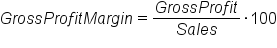Net Profit Margin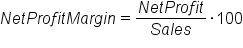Recall that gross profit is simply the revenue minus the cost of goods sold (COGS). Net profit is the gross profit minus all other expenses.

big idea
The difference between the two is that the gross profit margin shows the relationship between revenue and COGS, while the net profit margin shows the percentage of the money spent by customers that is turned into profit.

Companies need to have a positive profit margin in order to earn income, although having a negative profit margin may be advantageous in some instances (e.g., intentionally selling a new product below cost in order to gain market share).

This type of evaluation is typically used for internal evaluation. This is due to the difficulty that arises when trying to use this metric across multiple organizations. There is so much variation among businesses that it renders this metric less effective for external use. This ratio is helpful for evaluating the level of risk that exists in a sudden decline in sales. A lower profit margin indicates a higher susceptibility of danger in the event that sales decline rapidly.

terms to know

Profit Margin Ratio
A ratio that measures the amount of profit a company earns from its sales.
Gross Profit
The difference between net sales and the cost of goods sold.
Net Profit
Gross revenue minus all expenses.
```
```

## 3. Return on Assets (ROA) Ratio

The return on assets (ROA) ratio was developed by DuPont to show how effectively assets are being used. It is also a measure of how much the company relies on assets to generate profit. The higher the ratio, the better the company is at using its assets to generate income.

ROA can be broken down into multiple parts. The ROA is the product of two other common ratios – profit margin and asset turnover. When profit margin and asset turnover are multiplied together, the denominator of profit margin and the numerator of asset turnover cancel each other out, returning us to a ratio of net income to total assets.

formula

Return on Assets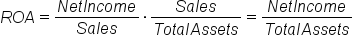Profit margin is net income divided by sales, measuring the percent of each dollar in sales that is profit for the company. Asset turnover is sales divided by total assets. This ratio measures how much each dollar in assets generates in sales. A higher ratio means that each dollar in assets produces more for the company.

ROA does have some drawbacks.

• It gives no indication of how the assets were financed. A company could have a high ROA, but still be in financial straits because all the assets were paid for through leveraging.
• The total assets are based on the carrying value of the assets, not the market value. If there is a large discrepancy between the carrying and market value of the assets, the ratio could provide misleading numbers.
• There is no metric to find a good or bad ROA. Companies that operate in capital intensive industries will tend to have lower ROAs than those who do not. The ROA is entirely contextual to the company, the industry, and the economic environment.
term to know

Return on Assets (ROA) Ratio
A ratio that measures how effectively assets are being used for generating profits.

## 4. Basic Earning Power (BEP) Ratio

Another profitability ratio is the basic earning power (BEP) ratio. The purpose of BEP is to determine how effectively a firm uses its assets to generate income. The higher the BEP ratio, the more effective a company is at generating income from its assets.

The BEP ratio is simply the earnings before interest and taxes (EBIT) divided by total assets.

formula

Basic Earning Power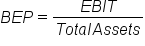This may seem remarkably similar to the return on assets ratio (ROA), which is operating income divided by total assets. EBIT, or earnings before interest and taxes, is a measure of how much money a company makes, but is not necessarily the same as operating income:

EBIT = Revenue – Operating expenses + Non-operating income
Operating income = Revenue – Operating expenses

The distinction between EBIT and Operating Income is non-operating income. Since EBIT includes non-operating income (such as dividends paid on the stock a company holds of another), it is a more inclusive way to measure the actual income of a company. However, in most cases, EBIT is relatively close to Operating Income.

The advantage of using EBIT, and thus BEP, is that it allows for more accurate comparisons of companies. BEP disregards different tax situations and degrees of financial leverage while still providing an idea of how good a company is at using its assets to generate income.

BEP, like all profitability ratios, does not provide a complete picture of which company is better or more attractive to investors. Investors should favor a company with a higher BEP over a company with a lower BEP because that means it extracts more value from its assets, but they still need to consider how things like leverage and tax rates affect the company.

terms to know

Basic Earning Power (BEP) Ratio
A ratio that measures how effectively a firm uses its assets to generate income.
EBIT
Earnings before interest and taxes; a measure of a business’s profitability.

## 5. Return on Equity (ROE) Ratio

Return on equity (ROE) ratio is a financial ratio that measures how good a company is at generating profit. ROE is the ratio of net income to equity. From the fundamental equation of accounting, we know that equity equals net assets minus net liabilities. Equity is the amount of ownership interest in the company and is commonly referred to as shareholders’ equity, shareholders’ funds, or shareholders’ capital.

In essence, ROE measures how efficient the company is at generating profits from the funds invested in it. A company with a high ROE does a good job of turning the capital invested in it into profit, and a company with a low ROE does a bad job. However, like many of the other ratios, there is no standard way to define a good ROE or a bad ROE. Higher ratios are better, but what counts as “good” varies by company, industry, and economic environment.

ROE can also be broken down into other components for easier use. ROE is the product of the net margin (profit margin), asset turnover, and financial leverage. Also note that the product of net margin and asset turnover is return on assets, so ROE is ROA times financial leverage.

formula

Return on Equity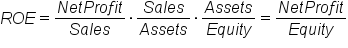Breaking ROE into parts allows us to understand how and why it changes over time.

• As profit margin goes up, each transaction brings in additional capital, thus increasing ROE.
• As total asset turnover goes up, the organization generates additional sales for each asset that is possessed, thus achieving a higher ROE.
• As financial leverage rises, so too does the taxable deduction, thus increasing ROE. Increased leverage does increase the risk and liability, so that is important to keep in mind.
term to know

Return on Equity (ROE) Ratio
A ratio that measures how effective a company is at using its equity to generate income.
summary
In this lesson, you learned about the various types of profitability ratios. The operating margin is a relatively simple measure of how profitable a company is from its operations, but it does not shed light on a company’s overall profitability. The profit margin ratio measures how much profit a company earns on its sales. The return on assets (ROA) ratio measures how much the company relies on assets to generate profit. The basic earning power (BEP) ratio determines how effectively a company uses its assets to generate income. Finally, return on equity (ROE) is the ratio of net income to equity, and measures how good a company is at generating profit.

Best of luck in your learning!

Source: THIS CONTENT HAS BEEN ADAPTED FROM LUMEN LEARNING'S “PROFITABILITY RATIOS” TUTORIAL.

Terms to Know
Basic Earning Power (BEP) Ratio

A ratio that measures how effectively a firm uses its assets to generate income.

EBIT

Earnings before interest and taxes; a measure of a business’s profitability.

Gross Profit

The difference between net sales and the cost of goods sold.

Net Profit

Gross revenue minus all expenses.

Operating Income

Equivalent to revenue minus operating expenses; does not include other expenses such as taxes and depreciation.

Operating Margin Ratio

A ratio that determines how much money a company is actually making in profit.

Profit Margin Ratio

A ratio that measures the amount of profit a company earns from its sales.

Return on Assets (ROA) Ratio

A ratio that measures how effectively assets are being used for generating profits.

Return on Equity (ROE) Ratio

A ratio that measures how effective a company is at using its equity to generate income.

Rating